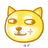V2EX = way to explore
V2EX 是一个关于分享和探索的地方

V2EX  ›  数据库

# 如何证明“Serializable Snapshot Isolation 能够保证 Serializability”？

JasonLaw · 39 天前 · 452 次点击

transaction 1 transaction 2
rr rr
rw rw
wr wr
ww ww

• r₁(x)r₁(x) & r₂(x)r₂(x) - 不管怎么并发执行，都可以得到和顺序执行一样的结果。
• r₁(x)r₁(x) & r₂(x)w₂(x) - SSI 允许 r₁(x)r₂(x)r₁(x)w₂(x)，而且它所执行的结果和 r₁(x)r₁(x)r₂(x)w₂(x)一样，所以保证了 Serializability ； SSI 避免了 r₁(x)r₂(x)w₂(x)r₁(x)的发生，因为 w₂(x)会使第一个 r₁(x)变得 stale，而且 r₁(x)r₂(x)w₂(x)r₁(x)无法得到和顺序执行一样的结果，所以保证了 Serializability 。
• r₁(x)r₁(x) & w₂(x)r₂(x) - SSI 避免了 r₁(x)w₂(x)r₁(x)r₂(x)的发生，因为 w₂(x)会使第一个 r₁(x)变得 stale，而且 r₁(x)w₂(x)r₁(x)r₂(x)无法得到和顺序执行一样的结果，所以保证了 Serializability 。r₁(x)w₂(x)r₂(x)r₁(x)同理。
• r₁(x)r₁(x) & w₂(x)w₂(x) - SSI 避免了 r₁(x)w₂(x)r₁(x)w₂(x)的发生，因为第一个 w₂(x)会使第一个 r₁(x)变得 stale，而且 r₁(x)w₂(x)r₁(x)w₂(x)无法得到和顺序执行一样的结果，所以保证了 Serializability 。r₁(x)w₂(x)w₂(x)r₁(x)同理。

1 条回复    2021-10-20 11:00:59 +08:001 zhengxiaowai   39 天前 分析的没错：SSI 保证 Serializability 就是排除掉异常情况我记得在工程实现上是用了一个什么图模型，然后判断是否有环，只有有环就是异常情况，直接取消事务这么来保证 Serializability 的